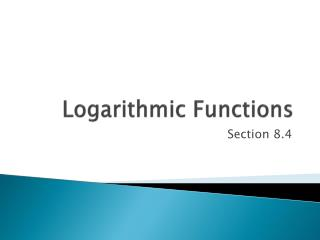DownloadDownload PresentationLogarithmic Functions

# Logarithmic Functions

Télécharger la présentation## Logarithmic Functions

- - - - - - - - - - - - - - - - - - - - - - - - - - - E N D - - - - - - - - - - - - - - - - - - - - - - - - - - -
##### Presentation Transcript

1. Logarithmic Functions Section 8.4

2. What is a logarithm? Logarithms were originally developed to simplify complex arithmetic calculations.

3. A logarithm is the power to which a number must be raised in order to get some other number • If y = bx, then log by = x • Read as “log base b of x” For example, the base ten logarithm of 100 is 2, because ten raised to the power of two is 100: 100 = 102because log 10100 = 2

4. The first, and perhaps the most important step, in understanding logarithms is to realize that they always relate back to exponential equations. • You must be able to convert an exponential equation into logarithmic form and vice versa.

5. Logarithm Form: y = logbx • Exponential form: by= x • Log is simply 3 letters used to denote dealing with a logarithm. Not variables or mult.

6. Examples: • 1. Write = 8 in log form • = 3 (“the log base 2 of 8 is 3”) 2. Write = 16 in log form = 2 3. Write = 1/8 in log form = -3

7. You try: • Write in log form • 1. = 49 = 2 • 2. = 1 = 0 • 3. = = -2 • 4. = 4 =

8. It is also very important to be able to start with a logarithmic expression and change this into exponential form. • This is simply the reverse of • what we just did.

9. Write in exponential form • 1. = 4 • = 81 • 2. = -3 • = 1/8 3. = 2 = 100 4. = -3 = 1/125

10. When working with logarithms, • if ever you get “stuck”, try • rewriting the problem in • exponential form. • Conversely, when working • with exponential expressions, • if ever you get “stuck”, try • rewriting the problem • in logarithmic form.

11. Exs. • 1. Solve for x: • = 2 • = x

12. 2. Solve for y: • = y • = • = • y = -2

13. 3. Evaluate: • =y • = 27 • = • y = 3

14. Common Logs • Let b be a positive real number such that b ≠ 1: • Logarithm of 1 = 0 b/c = 1 • Logarithm of base b = 1 b/c = b • Logarithm with base 10 is called common logarithm • = log x • Logarithm with base e is called natural logarithm • =

15. Evaluate • 1. • 2. • 3. • 4. • ( 4/3, -5/6, 3/2, 2 )

16. Graphs of Logarithmic Functions • A logarithmic function is the inverse of an exponential function Y = In other words, y= 10x and y=log10x are inverses of each other. Where is the line of reflection? Y =

17. Graphs cont. • The graph of y = + k has following characteristics: • The line x = h is a vertical asymptote • The domain is x > h and range is all real numbers • If b>1 , the graph moves up to the right. • If 0<b<1, the graph moves down to the right

18. Graph • 1. y = - 1 2. y =

19. Predict: what is the domain and range of y=log3(x-2) and y=log3(x)+1 • y=log3(x-2) y=log3(x)+1 Domain: x>0 Range: all real numbers Note: the +1 moves the parent graph up but does not affect the left or right translation, so the domain remains x>0 Domain: x>2 Range: all real numbers